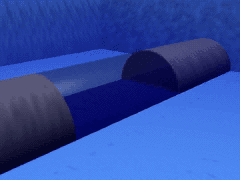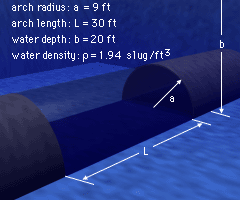Ch 7. Centroid/Distributed Loads/Inertia Multimedia Engineering Statics Centroid: Line Area Vol Centroid: Composite Distributed Loads Area Moment of Inertia
 Chapter 1. Basics 2. Vectors 3. Forces 4. Moments 5. Rigid Bodies 6. Structures 7. Centroids/Inertia 8. Internal Loads 9. Friction 10. Work & Energy Appendix Basic Math Units Sections Search eBooks Dynamics Fluids Math Mechanics Statics Thermodynamics Author(s): Kurt Gramoll ©Kurt GramollSTATICS - CASE STUDY IntroductionProblem Description An underwater archway is being added to a large aquarium so that visitors can view the fish up close. The water in the aquarium will exert a tremendous force downward onto the transparent arch. This force needs to be calculated accurately so that a safe design can be developed. What is known: The tunnel radius, a, of the glass arch is 9 ft. The water depth, b, is 20 ft. The length of the arch, L, is 30 ft. The water density ρ is 1.94 slug/ft3. QuestionDimension Diagram What is the resultant force exerted on the glass arch? Approach Determine the pressure exerted by the water on the surface of the arch. Integrate the x and y components of the pressure over the surface area of the transparent arch to get the resultant force in the x and the y directions respectively.

Practice Homework and Test problems now available in the 'Eng Statics' mobile app
Includes over 500 problems with complete detailed solutions.
Available now at the Google Play Store and Apple App Store.